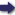(London :  Kegan Paul, Trench, Trübner & Co.,  1910.)

 Tools

## Search this bookPrev Page 30 Next``` 30 ALBERUNTS INDIA. dividing the product by 3,562,220, we get the partial unardtra days, viz., ii,455,224,575i;|-f-^;ffi. This sum of days without the fraction is subtracted from the partial lunar days, and the remainder, 720,635,951,963, represents the number of the civil days of our gauge-date. Dividing it by 7, we get as remainder 4, which means that the last of these days is a Wednesday. Therefore the Indian year commences with a Thursday. If we further want to find the adhimdsa time, we divide the adhimdsa days by 30, and the quotient is the number of the adhimdsas which have elapsed, viz. 727,661,633, plus a remainder of 28 days, 51 minutes, 30 seconds, for the current year. This is the time which has already elapsed of the adhimdsa month of the current year. To become a complete month, it only wants I day, 8 minutes, 30 seconds more. The same Wc havc here used the solar and lunar days, the calculation n ■ a i a • a , -i , n -i , '• applied to a aoAimasa and unaratra days, to find a certain past according'to portiou of a kalpa. We shall now do the same to find of Pulisa.^ the past portion of a caturyuga, and we may use the same elements for the computation of a caturyugct which we have used for that of a kalpa, for both methods lead to the same result, as long as we adhere to one and the same theory (e.g. that of Brahmagupta), and do not mix up different chronological systems, and as long as each gunakdra and its bhdgahhdra, which we here mention together, correspond to each other in the two computations. The former term means a m.ultiplicator in all kinds of calculations. In our (Arabic) astronomical hand¬ books, as well as those of the Persians, the word occurs in the form guncdr. The second term means each divisor. It occurs in the astronomical handbooks in the form hahcdr. It would be useless if we were to exemplify this com¬ putation on a caturyuga according to the theory of Brah- ```Prev Page 30 Next